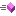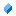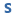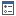﻿ NRange1DD Structure Members
Nevron .NET Vision
Nevron.GraphicsCore Namespace / NRange1DD Structure
Fields Properties Methods

In This Topic
NRange1DD Structure Members
In This Topic

The following tables list the members exposed by NRange1DD.

Public Constructors
NameDescriptionInitializer constructor
Top
Public Fields
NameDescriptionBegin valueRepresents the empty rangesEnd value
Top
Public Properties
NameDescriptionReturns true if the range is normal.
Top
Public Methods
NameDescriptionCombines the the two ranges.Overloaded. Returns true if the range contains the specified value.Returns true if the objects are equal.Tries to fit the passed range into this range.Gets the normalized begin valueGets the normalized end valueReturns the hash code of this instance.Returns the absolute lenght of this range.Returns the distance between the begin or end values of the range.Gets the range disposition of this range to another rangeGet the factor of the specified value relative to the range - 0 if value is equal to range.Begin and 1 if value is equal to range.EndReturns the nearest value that lies in this range.Inflates this range with the specified valueCalculates the intersection between two rangesChecks whether this range intersects with another range.Changes the begin and end values of this range.Returns true if the range is inverted.Normalizes the range.Translates the range
Top